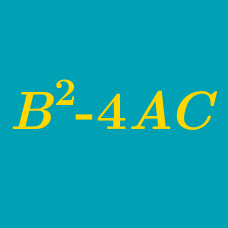Algebra

#### Challenge Quizzes

If $a = 2, b = 4, \text{ and } c = 1,$ what is the value of $b^2 - 4ac?$

If $a = -2, b = 3, \text{ and } c = -1,$ what is the value of $b^2 - 4ac?$

When solving with the quadratic formula, Scott gets $\sqrt{16 - 28}$ as part of his solution. The solutions will be:

A quadratic equation has a positive discriminant when set equal to zero. How many $x$-intercepts will the graph have?

What could be the value of $b$ so that a quadratic equation with a discriminant of $b^2 - 4(1)(2)$ has two real solutions?

×